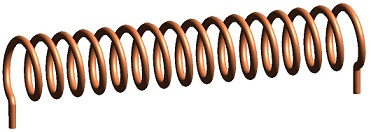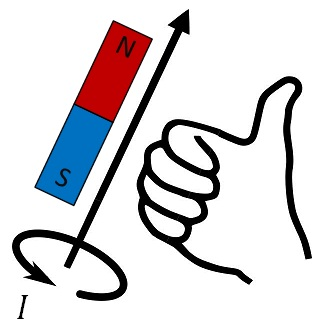# Difference Between Solenoid and Electromagnet

## Main Difference – Solenoid vs. Electromagnet

The terms solenoid and electromagnet are closely related. The main difference between solenoid and electromagnet is that solenoid is the name given to a long and narrow helical loop of wire, whereas an electromagnet is a magnet whose magnetic properties depend on an electric current. Most often, electromagnets are constructed using solenoids.

## What is a Solenoid

A solenoid refers to a wire coiled in a helical shape, as shown below:A solenoid

If the wire is long and thin, when a current is sent through the wire a uniform magnetic field forms inside the solenoid. The magnetic field strength$B$ for this uniform field can be given by:$B=\frac{\mu_0NI}{l}$

where$N$ is the number of turns in the coil,$I$ is the current and$L$ is the length of the solenoid.$\mu_0$ is the permeability of free space, given by$\mu_0=$ 1.26 × 10-6 m kg s-2 A-2. When the solenoid is palced in a material with a relative permeability$\mu_r$, the$\mu_0$ term is replaced by$\mu_0\mu_r$, where$\mu_r$ is the relative permeability of the material.

## What is an Electromagnet

An electromagnet is a magnet whose magnetic properties are produced by an electric current. When the current is switched off, the electromagnet no longer displays magnetic properties.

The simplest way to make an electromagnet is to make a solenoid, pass current through it and then place a piece of soft iron inside the solenoid. Due to the high relative permeability of soft iron, the magnetic field formed would be much stronger. Note that the wires need to be insulated: if they make direct contact with the iron core, the current will simply conduct through the iron core! Often, if you look at electromagnets found inside various appliances, it seems as though the copper wires are not insulated. However, in these cases they have been coated with an insulating material.

The rod of soft iron placed inside the solenoid would behave like an ordinary bar magnet when the current is switched on. The polarity of this electromagnet can be described by the right-hand grip rule. If you grip the solenoid so that your fingers curl around in the direction of current flow through the turns of the solenoid, your thumb will point to the “North” pole of the electromagnet.The right-hand grip rule

## Difference Between Solenoid and Electromagnet

### What it means

A solenoid is a long, thin helical loop of wire.

An electromagnet is a magnet whose magnetic properties depend on an electric current.

### Relationship

If a solenoid is made to conduct a current, it would become an electromagnet.

However, wires forming an electromagnet do not necessarily need to have a solenoid shape.

Image Courtesy

“A three-dimensional rendering of a solenoid.” by Zureks (Transferred from en.wikipedia to Commons) [Public Domain], via Wikimedia Commons

“The right-hand grip rule” by Right_hand_rule_simple.png: Original uploader was Schorschi2 at de.wikipedia [Public Domain], via Wikimedia Commons